Open in App
Not now

# Sieve of Eratosthenes

• Difficulty Level : Medium
• Last Updated : 15 Jan, 2023

Given a number n, print all primes smaller than or equal to n. It is also given that n is a small number.

Example:

Input : n =10
Output : 2 3 5 7

Input : n = 20
Output: 2 3 5 7 11 13 17 19

The sieve of Eratosthenes is one of the most efficient ways to find all primes smaller than n when n is smaller than 10 million or so (Ref Wiki).

Recommended Practice

Following is the algorithm to find all the prime numbers less than or equal to a given integer n by the Eratosthene’s method:
When the algorithm terminates, all the numbers in the list that are not marked are prime.

Explanation with Example:

Let us take an example when n = 50. So we need to print all prime numbers smaller than or equal to 50.

We create a list of all numbers from 2 to 50.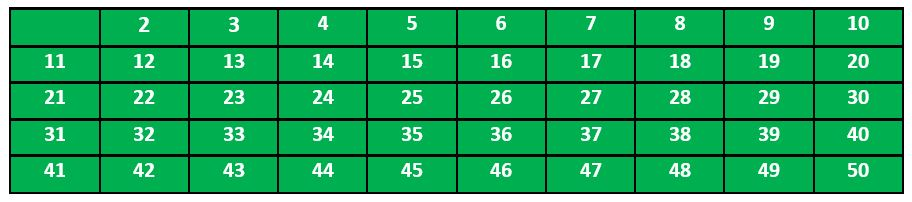According to the algorithm we will mark all the numbers which are divisible by 2 and are greater than or equal to the square of it.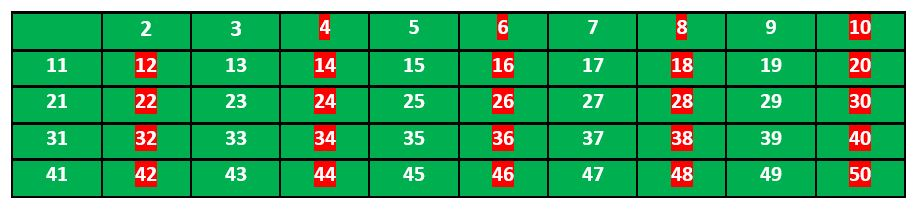Now we move to our next unmarked number 3 and mark all the numbers which are multiples of 3 and are greater than or equal to the square of it.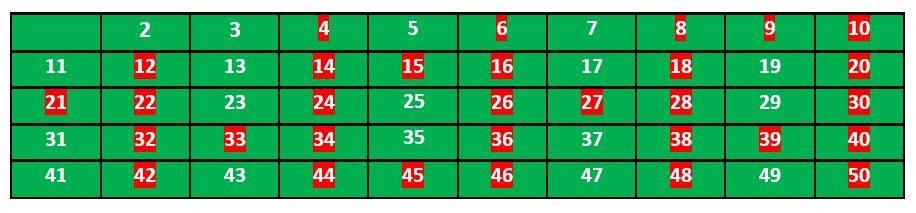We move to our next unmarked number 5 and mark all multiples of 5 and are greater than or equal to the square of it.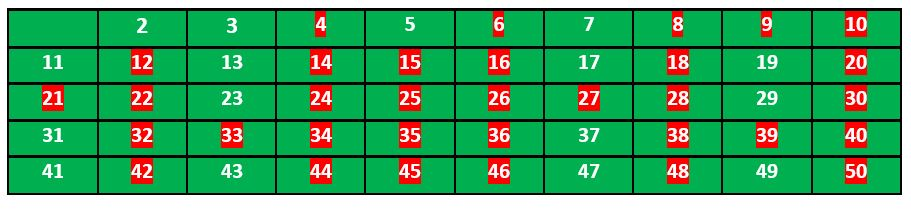We continue this process and our final table will look like below: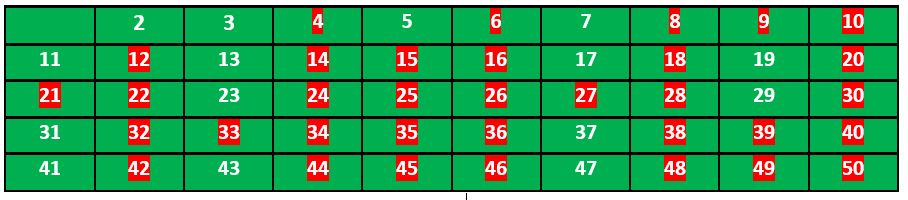So the prime numbers are the unmarked ones: 2, 3, 5, 7, 11, 13, 17, 19, 23, 29, 31, 37, 41, 43, 47.

Thanks to Krishan Kumar for providing the above explanation.

Implementation:

Following is the implementation of the above algorithm. In the following implementation, a boolean array arr[] of size n is used to mark multiples of prime numbers.

## C++

 `// C++ program to print all primes smaller than or equal to` `// n using Sieve of Eratosthenes` `#include ` `using` `namespace` `std;`   `void` `SieveOfEratosthenes(``int` `n)` `{` `    ``// Create a boolean array "prime[0..n]" and initialize` `    ``// all entries it as true. A value in prime[i] will` `    ``// finally be false if i is Not a prime, else true.` `    ``bool` `prime[n + 1];` `    ``memset``(prime, ``true``, ``sizeof``(prime));`   `    ``for` `(``int` `p = 2; p * p <= n; p++) {` `        ``// If prime[p] is not changed, then it is a prime` `        ``if` `(prime[p] == ``true``) {` `            ``// Update all multiples of p greater than or` `            ``// equal to the square of it numbers which are` `            ``// multiple of p and are less than p^2 are` `            ``// already been marked.` `            ``for` `(``int` `i = p * p; i <= n; i += p)` `                ``prime[i] = ``false``;` `        ``}` `    ``}`   `    ``// Print all prime numbers` `    ``for` `(``int` `p = 2; p <= n; p++)` `        ``if` `(prime[p])` `            ``cout << p << ``" "``;` `}`   `// Driver Code` `int` `main()` `{` `    ``int` `n = 30;` `    ``cout << ``"Following are the prime numbers smaller "` `         ``<< ``" than or equal to "` `<< n << endl;` `    ``SieveOfEratosthenes(n);` `    ``return` `0;` `}`

## C

 `// C program to print all primes smaller than or equal to` `// n using Sieve of Eratosthenes` `#include ` `#include ` `#include `   `void` `SieveOfEratosthenes(``int` `n)` `{` `  `  `    ``// Create a boolean array "prime[0..n]" and initialize` `    ``// all entries it as true. A value in prime[i] will` `    ``// finally be false if i is Not a prime, else true.` `    ``bool` `prime[n + 1];` `    ``memset``(prime, ``true``, ``sizeof``(prime));`   `    ``for` `(``int` `p = 2; p * p <= n; p++) {` `        ``// If prime[p] is not changed, then it is a prime` `        ``if` `(prime[p] == ``true``) {` `            ``// Update all multiples of p greater than or` `            ``// equal to the square of it numbers which are` `            ``// multiple of p and are less than p^2 are` `            ``// already been marked.` `            ``for` `(``int` `i = p * p; i <= n; i += p)` `                ``prime[i] = ``false``;` `        ``}` `    ``}`   `    ``// Print all prime numbers` `    ``for` `(``int` `p = 2; p <= n; p++)` `        ``if` `(prime[p])` `            ``printf``(``"%d "``,p);` `}`   `// Driver Code` `int` `main()` `{` `    ``int` `n = 30;` `    ``printf``(``"Following are the prime numbers smaller than or equal to %d \n"``, n);` `    ``SieveOfEratosthenes(n);` `    ``return` `0;` `}`   `// This code is contributed by Aditya Kumar (adityakumar129)`

## Java

 `// Java program to print all primes smaller than or equal to` `// n using Sieve of Eratosthenes`   `class` `SieveOfEratosthenes {` `    ``void` `sieveOfEratosthenes(``int` `n)` `    ``{` `        ``// Create a boolean array "prime[0..n]" and` `        ``// initialize all entries it as true. A value in` `        ``// prime[i] will finally be false if i is Not a` `        ``// prime, else true.` `        ``boolean` `prime[] = ``new` `boolean``[n + ``1``];` `        ``for` `(``int` `i = ``0``; i <= n; i++)` `            ``prime[i] = ``true``;`   `        ``for` `(``int` `p = ``2``; p * p <= n; p++) {` `            ``// If prime[p] is not changed, then it is a` `            ``// prime` `            ``if` `(prime[p] == ``true``) {` `                ``// Update all multiples of p greater than or` `                ``// equal to the square of it numbers which` `                ``// are multiple of p and are less than p^2` `                ``// are already been marked.` `                ``for` `(``int` `i = p * p; i <= n; i += p)` `                    ``prime[i] = ``false``;` `            ``}` `        ``}`   `        ``// Print all prime numbers` `        ``for` `(``int` `i = ``2``; i <= n; i++) {` `            ``if` `(prime[i] == ``true``)` `                ``System.out.print(i + ``" "``);` `        ``}` `    ``}`   `    ``// Driver Code` `    ``public` `static` `void` `main(String args[])` `    ``{` `        ``int` `n = ``30``;` `        ``System.out.print(``"Following are the prime numbers "``);` `        ``System.out.println(``"smaller than or equal to "` `+ n);` `        ``SieveOfEratosthenes g = ``new` `SieveOfEratosthenes();` `        ``g.sieveOfEratosthenes(n);` `    ``}` `}`   `// This code is contributed by Aditya Kumar (adityakumar129)`

## Python3

 `# Python program to print all` `# primes smaller than or equal to` `# n using Sieve of Eratosthenes`     `def` `SieveOfEratosthenes(n):`   `    ``# Create a boolean array` `    ``# "prime[0..n]" and initialize` `    ``#  all entries it as true.` `    ``# A value in prime[i] will` `    ``# finally be false if i is` `    ``# Not a prime, else true.` `    ``prime ``=` `[``True` `for` `i ``in` `range``(n``+``1``)]` `    ``p ``=` `2` `    ``while` `(p ``*` `p <``=` `n):`   `        ``# If prime[p] is not` `        ``# changed, then it is a prime` `        ``if` `(prime[p] ``=``=` `True``):`   `            ``# Update all multiples of p` `            ``for` `i ``in` `range``(p ``*` `p, n``+``1``, p):` `                ``prime[i] ``=` `False` `        ``p ``+``=` `1`   `    ``# Print all prime numbers` `    ``for` `p ``in` `range``(``2``, n``+``1``):` `        ``if` `prime[p]:` `            ``print``(p)`     `# Driver code` `if` `__name__ ``=``=` `'__main__'``:` `    ``n ``=` `20` `    ``print``(``"Following are the prime numbers smaller"``),` `    ``print``(``"than or equal to"``, n)` `    ``SieveOfEratosthenes(n)`

## C#

 `// C# program to print all primes` `// smaller than or equal to n` `// using Sieve of Eratosthenes` `using` `System;`   `namespace` `prime {` `public` `class` `GFG {`   `    ``public` `static` `void` `SieveOfEratosthenes(``int` `n)` `    ``{`   `        ``// Create a boolean array ` `        ``// "prime[0..n]" and` `        ``// initialize all entries` `        ``// it as true. A value in` `        ``// prime[i] will finally be ` `        ``// false if i is Not a` `        ``// prime, else true.`   `        ``bool``[] prime = ``new` `bool``[n + 1];`   `        ``for` `(``int` `i = 0; i <= n; i++)` `            ``prime[i] = ``true``;`   `        ``for` `(``int` `p = 2; p * p <= n; p++) ` `        ``{` `            ``// If prime[p] is not changed,` `            ``// then it is a prime` `            ``if` `(prime[p] == ``true``)` `            ``{` `                ``// Update all multiples of p` `                ``for` `(``int` `i = p * p; i <= n; i += p)` `                    ``prime[i] = ``false``;` `            ``}` `        ``}`   `        ``// Print all prime numbers` `        ``for` `(``int` `i = 2; i <= n; i++)` `        ``{` `            ``if` `(prime[i] == ``true``)` `                ``Console.Write(i + ``" "``);` `        ``}` `    ``}`   `    ``// Driver Code` `    ``public` `static` `void` `Main()` `    ``{` `        ``int` `n = 30;` `        ``Console.WriteLine(` `            ``"Following are the prime numbers"``);` `        ``Console.WriteLine(``"smaller than or equal to "` `+ n);` `        ``SieveOfEratosthenes(n);` `    ``}` `}` `}`   `// This code is contributed by Sam007.`

## PHP

 ``

## Javascript

 ``

Output

```Following are the prime numbers smaller  than or equal to 30
2 3 5 7 11 13 17 19 23 29 ```

Time Complexity: O(n*log(log(n)))
Auxiliary Space: O(n)

## C++

 `// the following implementation` `// stores only halves of odd numbers` `// the algorithm is a faster by some constant factors`   `#include ` `#include ` `using` `namespace` `std;`   `bitset<500001> Primes;` `void` `SieveOfEratosthenes(``int` `n)` `{` `    ``Primes = 1;` `    ``for` `(``int` `i = 3; i*i <= n; i += 2) {` `        ``if` `(Primes[i / 2] == 0) {` `            ``for` `(``int` `j = 3 * i; j <= n; j += 2 * i)` `                ``Primes[j / 2] = 1;` `        ``}` `    ``}` `}`   `int` `main()` `{` `    ``int` `n = 100;` `    ``SieveOfEratosthenes(n);` `    ``for` `(``int` `i = 1; i <= n; i++) {` `        ``if` `(i == 2)` `            ``cout << i << ``' '``;` `        ``else` `if` `(i % 2 == 1 && Primes[i / 2] == 0)` `            ``cout << i << ``' '``;` `    ``}` `    ``return` `0;` `}`

## Java

 `// Java program for the above approach` `import` `java.io.*;` `public` `class` `GFG {`   `  ``static` `int``[] Primes = ``new` `int``[``500001``];`   `  ``static` `void` `SieveOfEratosthenes(``int` `n)` `  ``{` `    ``Primes[``0``] = ``1``;` `    ``for` `(``int` `i = ``3``; i * i <= n; i += ``2``) {` `      ``if` `(Primes[i / ``2``] == ``0``) {` `        ``for` `(``int` `j = ``3` `* i; j <= n; j += ``2` `* i)` `          ``Primes[j / ``2``] = ``1``;` `      ``}` `    ``}` `  ``}`   `  ``// Driver Code` `  ``public` `static` `void` `main(String[] args)` `  ``{`   `    ``int` `n = ``100``;` `    ``SieveOfEratosthenes(n);` `    ``for` `(``int` `i = ``1``; i <= n; i++) {` `      ``if` `(i == ``2``)` `        ``System.out.print(i + ``" "``);` `      ``else` `if` `(i % ``2` `== ``1` `&& Primes[i / ``2``] == ``0``)` `        ``System.out.print(i + ``" "``);` `    ``}` `  ``}` `}`   `// This code is contributed by ukasp.`

## Python3

 `# Python program for the above approach` `Primes ``=` `[``0``] ``*` `500001` `def` `SieveOfEratosthenes(n) :` `    `  `    ``Primes[``0``] ``=` `1` `    ``i ``=` `3` `    ``while``(i``*``i <``=` `n) : ` `        ``if` `(Primes[i ``/``/` `2``] ``=``=` `0``) :` `            ``for` `j ``in` `range``(``3` `*` `i, n``+``1``, ``2` `*` `i) : ` `                ``Primes[j ``/``/` `2``] ``=` `1` `                `  `        ``i ``+``=` `2` `        `  `# Driver Code` `if` `__name__ ``=``=` `"__main__"``:`   `    ``n ``=` `100` `    ``SieveOfEratosthenes(n)` `    ``for` `i ``in` `range``(``1``, n``+``1``) :` `        ``if` `(i ``=``=` `2``) :` `            ``print``( i, end ``=` `" "``)` `        ``elif` `(i ``%` `2` `=``=` `1` `and` `Primes[i ``/``/` `2``] ``=``=` `0``) :` `            ``print``( i, end ``=` `" "``)` `    `  `    ``# This code is contributed by code_hunt.`

## C#

 `// C# program for the above approach` `using` `System;` `public` `class` `GFG {`   `  ``static` `int``[] Primes = ``new` `int``;`   `  ``static` `void` `SieveOfEratosthenes(``int` `n)` `  ``{` `    ``Primes = 1;` `    ``for` `(``int` `i = 3; i*i <= n; i += 2) {` `      ``if` `(Primes[i / 2] == 0) {` `        ``for` `(``int` `j = 3 * i; j <= n; j += 2 * i)` `          ``Primes[j / 2] = 1;` `      ``}` `    ``}` `  ``}`   `  ``// Driver Code` `  ``public` `static` `void` `Main(String[] args) {`   `    ``int` `n = 100;` `    ``SieveOfEratosthenes(n);` `    ``for` `(``int` `i = 1; i <= n; i++) {` `      ``if` `(i == 2)` `        ``Console.Write(i + ``" "``);` `      ``else` `if` `(i % 2 == 1 && Primes[i / 2] == 0)` `        ``Console.Write(i + ``" "``);` `    ``}` `  ``}` `}`   `// This code is contributed by sanjoy_62.`

## Javascript

 `// A JavaScript Program ` `// the following implementation` `// stores only halves of odd numbers` `// the algorithm is a faster by some constant factors`   `let Primes = ``new` `Array(500001).fill(0);`   `function` `SieveOfEratosthenes(n)` `{` `    ``Primes = 1;` `    ``for` `(let i = 3; i*i <= n; i += 2) {` `        ``if` `(Primes[Math.floor(i / 2)] == 0) {` `            ``for` `(let j = 3 * i; j <= n; j += 2 * i){` `                 ``Primes[Math.floor(j / 2)] = 1;` `            ``}` `        ``}` `    ``}` `}`   `let n = 100;` `SieveOfEratosthenes(n);` `let res = ``""``;` `for` `(let i = 1; i <= n; i++) {` `    ``if` `(i == 2){` `        ``res = res + i + ``" "``;` `    ``}` `    ``else` `if` `(i % 2 == 1 && Primes[Math.floor(i / 2)] == 0){` `        ``res = res + i + ``" "``;` `    ``}` `}` `console.log(res);`   `// The code is contributed by Gautam goel (gautamgoel962)`

Output

`2 3 5 7 11 13 17 19 23 29 31 37 41 43 47 53 59 61 67 71 73 79 83 89 97 `

Time Complexity: O(n*log(log(n)))
Auxiliary Space: O(n)

You may also like to see :

My Personal Notes arrow_drop_up
Related Articles